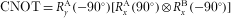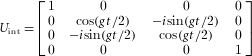## Main

Universal quantum gates are the key elements in a quantum computer, as they provide the fundamental building blocks for encoding complex algorithms and operations. Single-qubit rotations together with the two-qubit controlled-NOT (CNOT) are known to provide a universal set of gates12. Here, we present the complete characterization of a universal entangling gate, the square root of i-SWAP (SQiSW; ref. 13), from which gates such as the CNOT can be constructed. The pulse sequence for a CNOT can be written in terms of a SQiSW gate as follows:SQiSW RxA(180) SQiSW RyA(90), where Rjα(θ) is a rotation about axis j=x,y,z by an angle θ on qubit α=A,B. The SQiSW is a ‘natural’ two-qubit gate, as it directly results from capacitive coupling of superconducting qubits, yielding qubit coupling of the general form σAxσBx or σAyσBy, where σx,y are the Pauli spin operators for qubits A and B (refs 14, 15). Under the rotating-wave approximation, the corresponding interaction Hamiltonian has the form Hint=(g/2)(|01〉〈10|+|10〉〈01|), whereand g is the coupling strength that depends on design parameters.

When the two qubits are placed on-resonance, the two-qubit states are coupled by Hint as shown in Fig. 1a. The amplitudes of these states then oscillate in time, as described (in the rotating frame) by the unitary transformationwhere t is the interaction time and the representation is in the two-qubit basis set {|00〉,|01〉,|10〉,|11〉}. For an interaction time g t=π, the state amplitudes are swapped, such that |01〉→−i|10〉 and |10〉→−i|01〉. The SQiSW gate is formed by coupling for one-half this time, g t=π/2, producing cosine and sine matrix elements with equal magnitudes, thus entangling the qubits. When the qubits are off-resonance by an energy |Δ|g (Fig. 1b), the off-diagonal elements in Uint are small and have average amplitude g/Δ, effectively turning off the qubit–qubit interaction.

The electrical circuit for the capacitively coupled Josephson phase qubits16,17 used in this experiment is shown in Fig. 1c. Each phase qubit is a nonlinear resonator built from a Josephson inductance and an external shunting capacitance. When biased close to the critical current, the junction and its parallel loop inductance L give rise to a cubic potential with energy eigenstates that are unequally spaced. The two lowest levels are used as the qubit states |0〉 and |1〉, with transition frequency ω10. This frequency can be adjusted independently for each qubit through the bias current IbiasA,B. Each qubit’s state is detected through a single-shot measurement18,19, using a fast pulse IZA,B combined with read-out using an on-chip superconducting quantum interference device.

State preparation and tomography use single-qubit logic operations, corresponding to rotations about the x,y and z axes of the Bloch sphere19. Rotations about the z axis are produced by fast (nanosecond) current pulses IZA,B(t), which adiabatically change the qubit frequency, turning on and off the interaction and leading to phase accumulation between the |0〉 and |1〉 states. Rotations about any axis in the xy plane are produced by microwave pulses resonant with each qubit’s transition frequency, applied through IμwA,B(t). The phase of the microwave pulses defines the rotation axis in the xy plane, and the pulse duration and amplitude control the rotation angle. In previous work20, such single-qubit gates were shown to have fidelities of 98%, limited by the energy relaxation T1 and dephasing T2 times, which for this device were measured to be 400 and 120 ns, respectively.

The experimental design was chosen to give qubit frequencies ω10A,B/2π5.5 GHz. The strength of the coupling g=(Cc/C)ω10A,B was set by the coupling and qubit capacitances Cc≈2 fF and C≈1 pF, respectively. The coupling interaction is turned on and off by changing the relative qubit frequency Δ=ω10Aω10B through an adjustment of the qubit B bias IbiasB. A large detuning of Δoff/2π≈200 MHz was used to turn off the gate, yielding a small amplitude in the off-diagonal coupling g/Δoff≈0.055.

We first characterize the coupling by measuring the time dynamics of the entangling swap operationas shown in Fig. 2a. Initially, both qubits are tuned off-resonance by 200 MHz and allowed to relax to the |00〉 state. A π pulse on qubit A then produces the |10〉 state. A current pulse IZB(t) applied to IbiasB brings the qubits within a frequency Δ of resonance. After an interaction time tf, the bias IZB is reset to the original 200 MHz detuning and both qubits are then measured. Averaging over 1,200 events gives the probabilities for the four possible final states |00〉,|01〉,|10〉 and |11〉. The swapping behaviour for the states |01〉 and |10〉 as a function of tf is shown in Fig. 2b. On resonance (Δ=0), the swapping frequency between |01〉 and |10〉 gives an accurate measurement of the coupling strength g/2π=11 MHz.Figure 2: Characterization of coupling interaction and measurement of on/off ratio.

The amplitude of the swapping oscillations decreases with detuning as expected. In Fig. 2c we plot the peak-to-peak change in swap probability as a function of detuning Δ, compared to the theoretical prediction. Apart from a small reduction in the amplitude arising from imperfect measurement fidelity, the data is in good agreement with theory. At detunings |Δ|/2π>50 MHz, the swap amplitude is small and cannot be distinguished from the noise floor. From the maximum detuning bias of Δ/2π=200 MHz and from the coupling strength g/2π=11 MHz obtained from spectroscopy measurements and the data in Fig. 2b, we compute the probability ratio (Δ/g)2=(200/11)2=300 as a figure of merit for the on/off coupling ratio.

We fully characterize the SQiSW gate using QPT3,4. This involves preparing the qubits in a spanning set of input basis states, operating with the gate on this set of states, and then carrying out complete state tomography on the output. As illustrated in Fig. 3a, we first carry out quantum state tomography19,21 on the input state |01〉, which involves measuring the state along the x,y and z Bloch-sphere axes of each qubit, in nine separate experiments. We then operate on the |01〉 input state with SQiSW, and carry out complete state tomography on the output. These measurements allow for the evaluation of the two-qubit density matrix. This entire process is repeated 16 times in total, using four distinct input states for each qubit, chosen from the set {(|0〉,|1〉,|0〉+|1〉,|0〉+i|1〉)}. In Fig. 3b, we show the density matrix resulting from this tomography for one such input state:. From this complete set of measurements, we reconstruct the 16 by 16 χ matrix, the indices of which correspond to the Kronecker product of the operators {Ix,−iσyz} for each qubit3.Figure 3: Quantum state tomography for two sets of input and output states.

In a QPT experiment22,23,24 errors arise from the entangling gate and errors in measurement. As we are interested in the quality of the entangling gate itself, we have calibrated out errors resulting from measurement18. As described in the Supplementary Information, measurement errors arise from both a misidentification of the |0〉 and |1〉 states, and measurement crosstalk, where a measurement of |1〉 in one qubit increases the probability of a |1〉 measurement in the second qubit18. By carrying out further calibration experiments, we are able to determine the probabilities for these errors and correct the probabilities of the |00〉,|01〉,|10〉 and |11〉 final states.

In addition, standard QPT typically produces an unphysical χ matrix because of inherent experimental noise22,24. A physical χ matrix must be completely positive and trace preserving3 (CPTP), which implies it must have positive eigenvalues that sum to one. Our measured χ matrix has several negative eigenvalues, as discussed in the Supplementary Information. As is commonly done in QPT experiments22,24, a χ matrix that satisfies the CPTP constraints must be obtained from the experimental data before the data can be compared to a theoretically predicted χ matrix, which is physical by construction. This can be computed by noting that QPT is essentially analogous to system identification from classical control theory. More specifically, the problem of finding a physical approximation to unphysical QPT data can be shown to be a convex optimization problem25,26, a technique commonly used in control theory. We use a type of convex optimization called semidefinite programming25 to find the physical χ matrix that best approximates our measured, unphysical χ matrix. Mathematically, for the experimentally obtained χ matrix and the physical approximation χp, we minimize the two-norm distancewith the constraints that χp be CPTP.

This physical matrix χmp, which also includes the calibrations for measurement errors, is shown in Fig. 4. This matrix closely matches the original data before corrections for CPTP (see Supplementary Information). In both the real and imaginary parts of the χmp matrix, we observe non-zero matrix elements in locations where such elements are expected, in qualitative agreement with the theory, shown as the transparent bars. Quantitative comparison is obtained by calculating the process fidelity, 0<Fp<1, which gives a measure of how close χmp is to theoretical expectations24. For the SQiSW gate demonstrated here, with measurement calibration taken into account, we find Fp=Tr(χtχmp)=0.63, where χt is the theoretical χ matrix for the SQiSW gate. χm and χe, the unphysical χ matrices with and without measurement calibrations, respectively, and χep, the physical approximation to the χ matrix that does not include the measurement calibrations, are shown in Supplementary Figs S1–S3.Figure 4: Real and imaginary parts of the reconstructed χ matrix for the SQiSW gate, obtained from 16 possible input states .

Errors in our SQiSW gate primarily arise because the time for the experiment (50 ns) is not significantly shorter than the T2 dephasing time of 120 ns. This is confirmed using a recent theory5 by Kofman et al., which includes the effects of dephasing and decoherence on the SQiSW χ matrix. In particular, the elements marked with an asterisk and a circle in Fig. 4 are non-zero because of energy relaxation and dephasing, respectively. The modified Pauli basis used here gives a sign change in the real part of (χmp)X X,Y Y and (χmp)Y Y,X X, and the imaginary part of (χmp)X X,I I and (χmp)I I,X X. Using this theory and the real part of (χmp)I Z,I Z and (χmp)I Z,Z I, we estimate our single-qubit dephasing time as T2=(3π+2)/16g(χmp)I Z,I Z. From Fig. 4 we find (χmp)I Z,I Z=0.105 and T2=123 ns, in close agreement with the value mentioned above obtained from Ramsey experiments. We also estimate the degree of correlation of the dephasing noise between the coupled qubits using κ≈(χmp)I Z,Z I/(χmp)I Z,I Z−[(π−2)/(3π+2)]. Our measurement of (χmp)I Z,Z I≈0.017 yields, indicating that the dephasing is mostly uncorrelated. This is in agreement with previous work27,28,29 that found a dephasing mechanism local to the individual qubits.